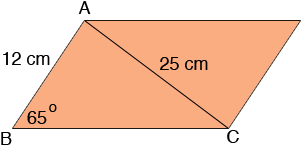SEARCH HOMEMath Central Quandaries & QueriesA parallelogram has one side that is 12.0 cm and one angle that is 65°. The shorter diagonal is 25.0 cm. To the nearest tenth of a centimeter, how long is the other side of the parallelogram? Use the sine law.Hi Felicia,The law of sines tells us that

sin(65o)/25 = sin(BCA)/12

Solve for sin(BCA) and then use the inverse sine function to find the measure of the angle BCA. Since the sum of the angles on a triangle is 180o you can then find the measure of the angle CAB. Now use the law of sines again to find the length of BC.

PennyMath Central is supported by the University of Regina and The Pacific Institute for the Mathematical Sciences.